# Consecutive numbers

Sum of ten consecutive numbers is 105. Determine these numbers (write first and last).

a1 =  6
a10 =  15

### Step-by-step explanation: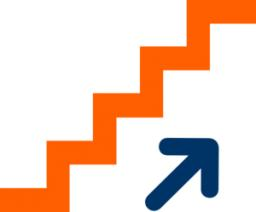Did you find an error or inaccuracy? Feel free to write us. Thank you!## Related math problems and questions:

• Find the sumFind the sum of all natural numbers from 1 and 100, which are divisible by 2 or 5
• Sum of the seventeen numbersThe sum of the 17 different natural numbers is 154. Determine the sum of the two largest ones.
• Three ints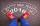The sum of three consecutive integers is 2016. What numbers are they?
• Sequence 3Write the first 5 members of an arithmetic sequence: a4=-35, a11=-105.
• AM of three numbersThe number 2010 can be written as the sum of 3 consecutive natural numbers. Determine the arithmetic mean of these numbers.
• Find twoFind two consecutive natural numbers whose product is 1 larger than their sum. Searched numbers expressed by a fraction whose numerator is the difference between these numbers and the denominator is their sum.
• The sumThe sum of the first 10 members of the arithmetic sequence is 120. What will be the sum if the difference is reduced by 2?
• Finite arithmetic sequenceHow many numbers should be inserted between the numbers 1 and 25 so that all numbers create a finite arithmetic sequence and that the sum of all members of this group is 117?
• In the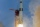In the arithmetic sequence a1 = 4.8, d = 0.4. How many consecutive members, starting with the first, need to be added so that the sum is greater than 170?
• Sum of membersWhat is the sum of the first two members of the aritmetic progression if d = -4.3 and a3 = 7.5?
• Three numbers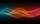The sum of the three numbers is 287. These numbers are in a ratio of 3: 7: 1/4. Find these numbers.
• Three unknown numbers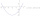The sum of of three numbers is 106. the first number is 10 more then the third. The second number is 2x the third. What are the numbers?
• DigitsShow that if x, y, z are 3 consecutive nonzero digits, zyx-xyz = 198, where zyx and xyz are three-digit numbers created from x, y, z.
• Sum of moneyOn a certain sum of money, invested at the rate of 10% compounded annually, the difference between the interests of the first year and the third year is 315 . Find the sum.
• Have solutionThe sum of four consecutive even numbers is 92. Determine these numbers.
• Two geometric progressions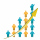Insert several numbers between numbers 6 and 384 so that they form with the given GP numbers and that the following applies: a) the sum of all numbers is 510 And for another GP to apply: b) the sum of entered numbers is -132 (These are two different geome
• Sequence 2Write the first 5 members of an arithmetic sequence a11=-14, d=-1